#### Starting from a look-at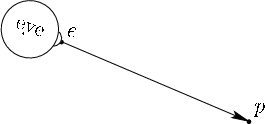For VR, the position and orientation of the eye in the virtual world are given by a tracking system and possibly controller inputs. By contrast, in computer graphics, it is common to start with a description of where the eye is located and which way it is looking. This is called a look-at, and has the following components:

1. Position of the eye: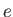2. Central looking direction of the eye: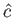3. Up direction: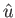.
Bothandare unit vectors. The first directioncorresponds to the center of the view. Whateveris pointing at should end up in the center of the display. If we want this to be a particular point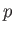in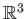(see Figure 3.15), thencan be calculated as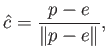(3.37)

in which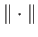denotes the length of a vector. The result is just the vector fromto, but normalized.

The second directionindicates which way is up. Imagine holding a camera out as if you are about to take a photo and then performing a roll rotation. You can make level ground appear to be slanted or even upside down in the picture. Thus,indicates the up direction for the virtual camera or eye.

We now construct the resulting transform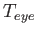from (3.36). The translation components are already determined by, which was given in the look-at. We need only to determine the rotation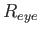, as expressed in (3.35). Recall from Section 3.2 that the matrix columns indicate how the coordinate axes are transformed by the matrix (refer to (3.7) and (3.8)). This simplifies the problem of determining. Each column vector is calculated as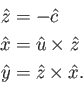(3.38)

The minus sign appears for calculating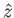because the eye is looking down the negative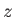axis. The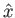direction is calculated using the standard cross product. For the third equation, we could use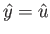; however,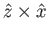will cleverly correct cases in whichgenerally points upward but is not perpendicular to. The unit vectors from (3.38) are substituted into (3.35) to obtain. Thus, we have all the required information to construct.

Steven M LaValle 2020-11-11